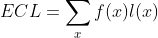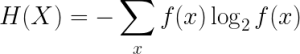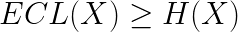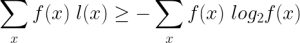# Anytime Algorithm For Machine Learning

For example, to encode 2 numbers, we need codes of 1-bit length, which are ‘0’ and ‘1’.

To encode 4 = 2^2 numbers, we need codes of 2-bits length, which are: ’00’, ’01’, ’10’, ’11’

To encode 256 = 2^8 numbers, we need codes of 8-bits length, which are: … [we skip it :)]

Consider one radar image of dimension 401×401 including float numbers that take value from 0 to 200 mm/h. If we are interested in one decimal number, this data can be multipled by 10 and saved as interger. At that time, the array contains 401×401 intergers, whose values are from 0 to 2000.

Following the above logic, to encode 2000 different numbers, we need 11-bits codes, because 2^11 = 2048. If so, the total number of bits to encode this data is: 401 x 401 x 11 bits = 1,768,811 bits.

Compressing data aims to reduce the total number of bits that are used to encode it, in other words, to find an encoder that has a minimum average of code lengths.

## Expected code length

Expected or average code length (ECL) of a data d is defined as:where x is values of data, f(x) is its frequency, and l(x) is the length of code used to encode the value x. This quantity tells us how many of bits on average is used to encode a number in the data x and by a Code C.

## Minimum expected code length

It can be proved mathematically that, the ECL of a code is bounded below by the entropy of given data. What is entropy of a data? It’s a quantity to measure information, that was initially introduced by Shannon (a Mathematician and father of Information Theory). This quantity tells us how much uncertainty the data contains.

In mathematical point of view, the entropy of the data is defined asAnd by the source coding theorem , the following inequality is always truewhich is equivalent toThe ECL achieves the minimum value if l(x) = -log2 [f(x)], meaning that, the length of the code of value x depends on the frequency of that value in the data. For example, in our radar images, the most frequent number is 0 (0.1mm/h), then we should use a short string of bits to encode the value 0. On the contrary, the number 2000 (0.1mm/h) has a very low frequency, then a longer bit-string must be used. In that way, the average of bits used to encode one value will be reduced to the entropy value, which is the lower bound of the compression. If we use a code which yields an ECL less than the entropy, then the compression makes loss of information.

In summary, the compressing could be minimized if the length of codes follows the formula: l(x) = -log2 [f(x)].

## The Huffman Encoding

The Huffman algorithm is one of methods to encode data into bit-strings whose ECL approximates to the lower bound of compression. This is a very simple algorithm that can be produced by a several code lines in Python. For detailed of Huffman algorithm, you can refers to the link: